# 基于精确准直线阵探测器的康普顿背散射成像仿真Compton Backscatter Imaging Simulation Based on Precise Collimated Linear Array Detector

DOI: 10.12677/APP.2021.112015, PDF, HTML, XML, 下载: 84  浏览: 509

Abstract: Conventional Compton backscatter imaging image reconstruction methods include precise focusing and energy spectrum analysis. Accurate focusing requires point-by-point scanning, long scanning time and low ray utilization; energy spectrum analysis relies on high-time resolution detectors, which can obtain limited energy spectrum data, and the reconstruction algorithm has high computational complexity and time-consuming. Therefore, this paper proposes a Compton backscatter imaging system based on a linear array detector on the premise of a precise focusing method. A collimator is installed on each detection unit of the ray source and the linear array detector to determine the incident source and the linear array. After the spatial position of the detector, the linear array detector can only receive backscattered photons from a fixed scattering angle and with a fixed energy, and the object to be measured is divided into several unit blocks. Each unit block is scanned, and each time the linear array detector is scanned, the depth-photon number information of the corresponding unit block can be obtained to complete the backscattered photon positioning. In the subsequent image reconstruction process, the ray attenuation is considered, and the attenuation correction can be completed by using only the backscattered photon information collected by the linear array detector without using the energy spectrum data, and the electron density of each point inside the object to be measured is obtained to obtain the reconstructed image. This paper uses the Monte Carlo method to establish a simulation model to complete the simulation of the Compton backscatter imaging process. After theoretical calculation, the attenuation correction is completed, and the electron density of each point in the object and the reconstructed image are obtained.

1. 引言

2. 康普顿散射

2.1. 康普顿散射原理

X射线通过实物物质发生散射时，散射光中除了有原波长λ0的X射线外，还产生了波长λ大于λ0的X射线，其波长的增量随散射角的不同而变化。这种现象称为康普顿散射，亦称康普顿效应。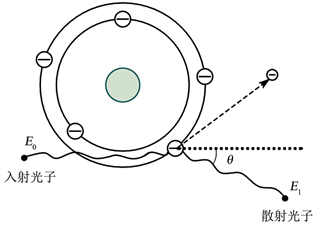Figure 1. Compton scattering diagram

$\Delta \lambda =\lambda -{\lambda }_{0}=\frac{c}{\nu }-\frac{c}{{\nu }_{0}}=\frac{h}{{m}_{0}c}\left(1-\mathrm{cos}\theta \right)$ (1)

${E}_{1}=\frac{{E}_{0}}{1+\alpha \left(1-\mathrm{cos}\theta \right)}$ (2)

$\alpha =\frac{h\upsilon }{{m}_{0}{c}^{2}}=\frac{h}{{m}_{0}c}×\frac{\upsilon }{c}=\frac{{\lambda }_{e}}{\lambda }$

2.2. 康普顿微分散射截面

$\begin{array}{l}\frac{\text{d}\sigma \left({E}_{0},\theta \right)}{\text{d}\Omega }=\frac{{r}_{e}^{2}}{2}\left(\frac{{E}_{0}}{{E}_{1}}+\frac{{E}_{1}}{{E}_{0}}-{\mathrm{sin}}^{2}\theta \right){\left(\frac{{E}_{1}}{{E}_{0}}\right)}^{2}\\ =\frac{{r}_{e}^{2}}{2}\left[{\mathrm{cos}}^{2}\theta +\alpha \left(1-\mathrm{cos}\theta \right)+\frac{1}{1+\alpha \left(1-\mathrm{cos}\theta \right)}\right]{\left[\frac{1}{1+\alpha \left(1-\mathrm{cos}\theta \right)}\right]}^{2}\\ ={r}_{e}^{2}{\left[\frac{1}{1+\alpha \left(1-\mathrm{cos}\theta \right)}\right]}^{2}\left[\frac{1+{\mathrm{cos}}^{2}\theta }{2}\right]\left[1+\frac{{\alpha }^{2}{\left(1-\mathrm{cos}\theta \right)}^{2}}{\left(1+{\mathrm{cos}}^{2}\theta \right)\left[1+\alpha \left(1-\mathrm{cos}\theta \right)\right]}\right]\end{array}$ (3)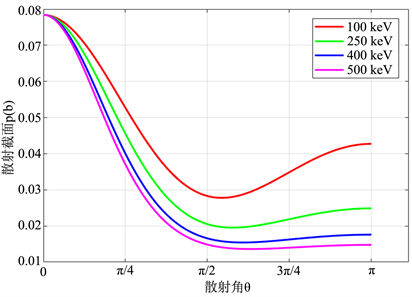Figure 2. The relationship of differential scattering cross section with incident photon energy and scattering angle

2.3. 射线衰减校正

$\mu ={\mu }_{光电效应}+{\mu }_{康普顿效应}+{\mu }_{电子对效应}$ (4)

${\mu }_{康普顿效应}=\sigma \left(E\right)\cdot \frac{{N}_{A}\cdot \rho }{A}$ (5)

$\sigma \left(E\right)$ 为单个原子的康普顿散射截面，有 ：

$\sigma \left(E\right)=Z\cdot {\sigma }_{c}\left(E\right)$ (6)

${\sigma }_{c}\left(E\right)$ 为单个电子的康普顿散射截面，是单个电子的康普顿微分散射截面对整个立体角的积分：

${\sigma }_{c}=2\pi {r}_{e}^{2}\left\{\frac{1+\alpha }{{\alpha }^{2}}\left[\frac{2\left(1+\alpha \right)}{1+2\alpha }-\frac{\mathrm{ln}\left(1+2\alpha \right)}{\alpha }\right]+\frac{\mathrm{ln}\left(1+2\alpha \right)}{2\alpha }-\frac{1+3\alpha }{{\left(1+2\alpha \right)}^{2}}\right\}$ (7)

$\alpha =\frac{E}{\text{2}\pi {m}_{0}{c}^{2}}$

${\mu }_{康普顿效应}={\sigma }_{c}\left(E\right)\cdot \frac{{N}_{A}\cdot \rho }{A}\cdot Z$ (8)

${\rho }_{e}=\rho \cdot {N}_{A}\cdot \frac{Z}{A}$ (9)

${\mu }_{康普顿效应}={\sigma }_{c}\left(E\right)\cdot {\rho }_{e}$ (10)

$n={n}_{0}×\frac{\text{d}\sigma }{\text{d}\Omega }\left({E}_{0},\theta \right)×\text{d}V×\text{d}\Omega ×{\rho }_{e}×{f}_{i}\left({E}_{0}\right)×{f}_{s}\left({E}_{1}\right)×k$ (11)

$\begin{array}{l}{f}_{i}\left({E}_{0}\right)={\text{e}}^{-{\int }_{a}^{r}\mu \left(l\right)\text{d}l}\\ {f}_{s}\left({E}_{1}\right)={\text{e}}^{-{\int }_{r}^{b}\mu \left(l\right)\text{d}l}\end{array}$

n为接收到的背散射光子数；n0为入射光子数； $\frac{\text{d}\sigma }{\text{d}\Omega }\left({E}_{0},\theta \right)$ 为康普顿微分散射截面； $\text{d}V=\text{d}S\ast \text{d}z$ 代表着单次测量的有效体积，dS为射线束的横截面积，dz是入射线方向上的一个线元；dΩ是探测器对散射点所张的立体角； ${f}_{i}\left({E}_{0}\right)$ 代表入射线到达散射点前的入射衰减、 ${f}_{s}\left({E}_{1}\right)$ 代表散射点到达探测器前的出射衰减，其中 $\mu \left(l\right)$ 为线性衰减系数值；k为探测器的探测效率。

$n={n}_{0}\cdot k\cdot \frac{\text{d}\sigma }{\text{d}\Omega }\left({E}_{0},\theta \right)\cdot \text{d}S\cdot \text{d}z\cdot \text{d}\Omega \cdot {\rho }_{e}\cdot \mathrm{exp}\left(-{\int }_{A}^{X}{\rho }_{e}\cdot {\sigma }_{c}\left({E}_{0}\right)\text{d}l\right)\cdot \mathrm{exp}\left(-{\int }_{X}^{C}{\rho }_{e}\cdot {\sigma }_{c}\left({E}_{\text{1}}\right)\text{d}l\right)$ (12)

${\rho }_{e}\cdot \mathrm{exp}\left(-{\int }_{A}^{X}{\rho }_{e}\cdot {\sigma }_{c}\left({E}_{0}\right)\text{d}l\right)\cdot \mathrm{exp}\left(-{\int }_{X}^{C}{\rho }_{e}\cdot {\sigma }_{c}\left({E}_{\text{1}}\right)\text{d}l\right)=\frac{n}{{n}_{0}\cdot k\cdot \frac{\text{d}\sigma }{\text{d}\Omega }\left({E}_{0},\theta \right)\cdot \text{d}S\cdot \text{d}z\cdot \text{d}\Omega }$ (13)

dl为入射线方向上的一个线元，将衰减系数的表达式中的积分变成求和后，dl不再是趋于无限小，而是一个可根据探测单元尺寸求出的定值Δl，这样就把求解每个散射点的电子密度变成求解每个散射单元的平均电子密度的问题了。

$\begin{array}{l}{\int }_{A}^{X}{\rho }_{e}\cdot {\sigma }_{c}\left({E}_{0}\right)\text{d}l\approx {\sigma }_{c}\left({E}_{0}\right)\cdot \underset{i=1}{\overset{n}{\sum }}{\rho }_{ei}\\ {\int }_{X}^{C}{\rho }_{e}\cdot {\sigma }_{c}\left({E}_{1}\right)\text{d}l\approx {\sigma }_{c}\left({E}_{1}\right)\cdot \underset{i=1}{\overset{m}{\sum }}{\rho }_{ei}\end{array}$ (14)

${\rho }_{e}\cdot \mathrm{exp}\left(-{\sigma }_{c}\left({E}_{0}\right)\cdot \underset{i=1}{\overset{n}{\sum }}{\rho }_{ei}\right)\cdot \mathrm{exp}\left(-{\sigma }_{c}\left({E}_{1}\right)\cdot \underset{j=\text{0}}{\overset{m}{\sum }}{\rho }_{ej}\right)=\frac{n}{{n}_{0}\cdot k\cdot \frac{\text{d}\sigma }{\text{d}\Omega }\left({E}_{0},\theta \right)\cdot \text{d}S\cdot \text{d}z\cdot \text{d}\Omega }$ (15)

$\begin{array}{l}{\rho }_{e}\cdot \mathrm{exp}\left(-{\sigma }_{c}\left({E}_{0}\right)\cdot \underset{i=1}{\overset{n\text{-1}}{\sum }}{\rho }_{ei}-{\sigma }_{c}\left({E}_{0}\right)\cdot {\rho }_{e}\right)\cdot \mathrm{exp}\left(-{\sigma }_{c}\left({E}_{1}\right)\cdot {\rho }_{e}-{\sigma }_{c}\left({E}_{1}\right)\cdot \underset{j=1}{\overset{m}{\sum }}{\rho }_{ej}\right)\\ =\frac{n}{{n}_{0}\cdot k\cdot \frac{\text{d}\sigma }{\text{d}\Omega }\left({E}_{0},\theta \right)\cdot \text{d}S\cdot \text{d}z\cdot \text{d}\Omega }\end{array}$ (16)

$\begin{array}{l}{\rho }_{e}\cdot \mathrm{exp}\left\{-\left[{\sigma }_{c}\left({E}_{0}\right)+{\sigma }_{c}\left({E}_{1}\right)\right]\cdot {\rho }_{e}\right\}\cdot \mathrm{exp}\left\{-\left[{\sigma }_{c}\left({E}_{0}\right)\cdot \underset{i=1}{\overset{n\text{-1}}{\sum }}{\rho }_{ei}+{\sigma }_{c}\left({E}_{1}\right)\cdot \underset{j=1}{\overset{m}{\sum }}{\rho }_{ej}\right]\right\}\\ =\frac{n}{{n}_{0}\cdot k\cdot \frac{\text{d}\sigma }{\text{d}\Omega }\left({E}_{0},\theta \right)\cdot \text{d}S\cdot \text{d}z\cdot \text{d}\Omega }\end{array}$ (17)

3. 康普顿背散射成像模型

X射线源发射X射线至待测物体，根据待测物体的xyz空间中的坐标范围可构建一个宽高长为x1, y1, z1的长方体，示意图如图3所示，将长方体在xoy面分解成m × n个 $\frac{{x}_{1}}{m}×\frac{{y}_{1}}{n}×{z}_{1}$ 的单元块，对每个单元块进行一次扫描(确定射线源和探测器与长方体的空间夹角不变，整体在xoy面平移)，总计m × n次扫描，即可完成对待测物体的深度–背散射光子数信息的获取。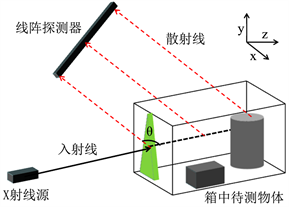Figure 3. Schematic diagram of Compton backscatter imaging system

4. 蒙特卡罗模拟计算

4.1. 基于Geant4的蒙特卡洛模拟

Geant4 (Geometry And Tracking)是由欧洲核子中心(CERN)开发的一套C++开源工具包，其用途是模拟粒子在物质中的物理过程。Geant4由于C++强大的语言能力，因而能够灵活地处理规模更加庞大、结构更加复杂的物理环境。Geant4几乎可以模拟所有物理过程，包括电磁相互作用、中子散射、光学过程等。除了在高能物理方面的应用，Geant4还被广泛应用于核物理、空间和天体物理、医用物理、辐射防护和探测等。

4.2. 在Geant4中的仿真模型的建立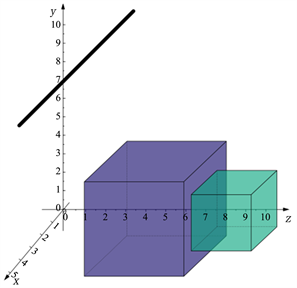(a) 实验物理模型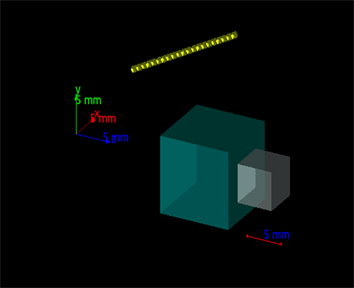(b) Geant4中仿真模型

Figure 4. Simulation model diagram

4.3. 仿真结果与分析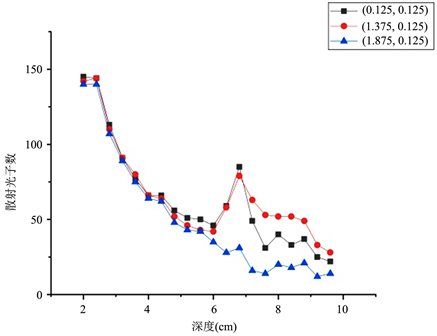Figure 5. Depth-scattering photon numbers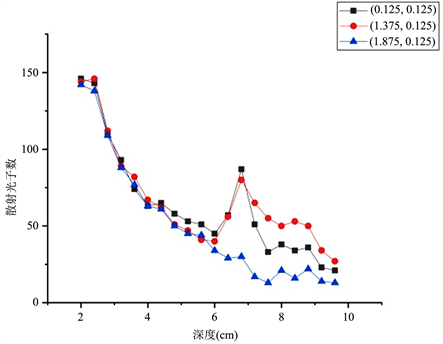Figure 6. Depth-scattering photon numbers of confirmatory experiment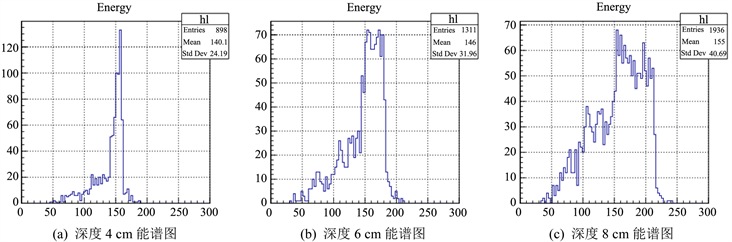Figure 7. Energy spectrum at different depths of (0.125, 0.125) unit block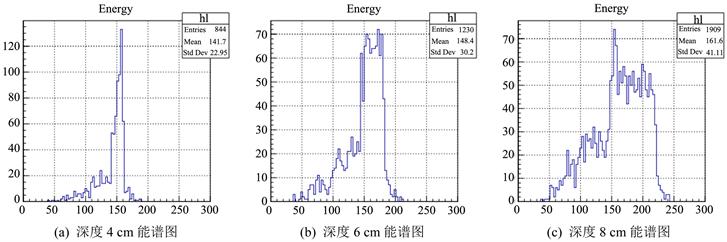Figure 8. Energy spectrum at different depths of (1.375, 0.125) unit block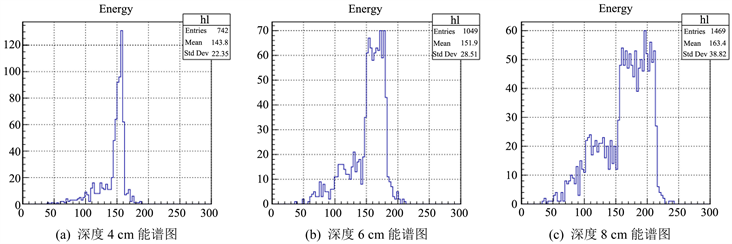Figure 9. Energy spectrum at different depths of (1.875, 0.125) unit block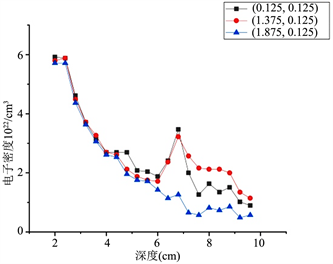Figure 10. Depth-uncorrected electron density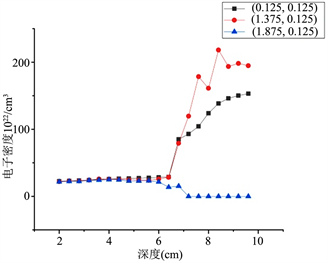Figure 11. Depth-corrected electron densityFigure 12. Image without attenuation correction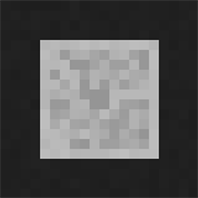Figure 13. Image after attenuation correctionFigure 14. Image without attenuation correction after stretchingFigure 15. Attenuation correction image after stretchingTable 1. Image quality evaluation resultsFigure 16. Virtual original

5. 结论

NOTES

*通讯作者。

  包尚联. 现代医学影像物理学[M]. 北京: 北京大学医学出版社, 2004.  崔玉华. X射线康普顿散射成像技术的研究与应用[J]. 中国安防, 2012(3): 41-44.  郑玉来, 王强. 康普顿背散射包裹检测的蒙特卡罗模拟[[J]. 中国原子能科学研究院年报, 2010(1): 418-420.  Tarpau, C., Cebeiro, J., Nguyen, M.K., et al. (2020) Analytic In-version of a Radon Transform on Double Circular Arcs with Applications in Compton Scattering Tomography. IEEE Transactions on Computational Imaging, PP, 1. https://doi.org/10.1109/TCI.2020.2999672  王加俊, 黄贤武, 仲兴荣. 完全最小二乘下的康普顿散射图像重建[J]. 仪器仪表学报, 2004, 25(2): 164-167.  许淑艳. 蒙特卡罗方法在实验核物理中的应用[M]. 北京: 原子能出版社, 2006.  丁富荣, 班勇, 夏宗璜. 辐射物理[M]. 北京: 北京大学出版社, 2004.  Alenezi, M., Stinson, K.R., Maqbool, M., et al. (2018) Klein-Nishina Electronic Cross-Section, Compton Cross Sections, and Buildup Factor of Wax for Radiation Shielding and Protection. Journal of Radiological Protection, 38, 372-381. https://doi.org/10.1088/1361-6498/aaa57b  Dyson, N.A. (1990) X-Rays in Atomic and Nuclear Physics. Cam-bridge University Press, Cambridge. https://doi.org/10.1017/CBO9780511470806  Thorsten, M.B. (2008) Computed Tomography. Springer, Ger-many.  董文斌. 利用康普顿背散射的蒙特卡罗模拟分析物质组成的研究[D]: [硕士学位论文]. 长春: 吉林大学, 2008.  古宇飞. 基于能谱解析的康普顿背散射成像重建算法研究[D]: [硕士学位论文]. 郑州: 解放军信息工程大学, 2014  Webber, J.W. and Lionheart, W.R.B. (2018) Three Dimensional Compton Scattering To-mography. Inverse Problems, 34, Article ID: 084001. https://doi.org/10.1088/1361-6420/aac51e  梁金昆. 关于康普顿效应的两个问题[J]. 无损检测, 1999, 21(1): 39-41.